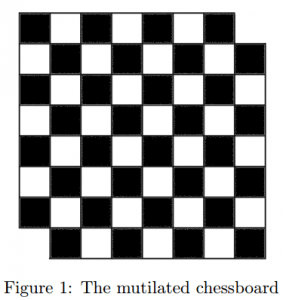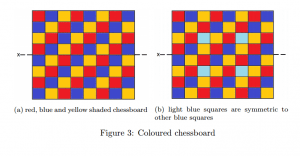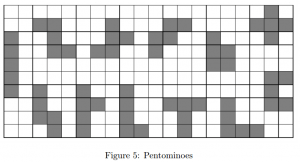# How to tile a chessboard

Introducing polyominoesTetris is a well-known Russian tile-matching game released in 1984. The pieces in Tetris, known as Tetriminos, contain 4 squares each connected by the edges and are based on tetrominoes, the 4-square specific version of a polyomino. This term ‘polyomino’ was coined by Solomon Golomb when he was still a 22 year old graduate student at Harvard in an article published in the American Mathematical Monthly.

Golomb begins by explaining the mutilated chessboard. Imagine a chessboard with the two corner tiles removed (Figure 1). Would you be able to cover the chessboard with dominoes, where each domino covers two squares of the chessboard with no overlap? Despite the chessboard having an even number of squares the answer is ‘no’. With the chessboard coloured in the usual way each domino would cover one white and one black square. Thus $\it{n}$ dominoes would cover $\it{n}$ white and $\it{n}$ black squares. But the chessboard has more black than white squares since two white squares were removed and so cannot be covered with dominoes.The domino is the 2-square specific version of a polyomino. Figure 2 shows nine varieties of polyomonioes. The tetrominoes in this figure will be familiar to anyone who has played tetris.Going back to the task of covering an 8×8 chessboard, we could try covering it with 21 trominoes and 1 monomino. First try covering the chessboard using 21 straight trominoes and one monomino in the upper left corner. You will find that this is not possible. Golomb explains this through the use of colour. If we were to colour in the squares of the chessboard in red, blue and yellow as shown in figure 3a we would see that there are 21 red, 22 blue and 21 yellow coloured squares. Each straight tromino would cover one of each colour. But the upper left corner is red so by covering it with a monomino we would be left with 20 red, 22 blue and 21 yellow squares. Covering the board with straight trominoes and one monomino in the upper left corner is impossible as these are unequal. The same argument can be used if we were to cover any red or yellow square with the monomino. We now recognise that the monomino must cover a blue square. Covering the left corner with the monomino however, gives us the same problem as covering the upper left corner due to symmetry in the $\it{x}$-axis. We find that only blue squares which are symmetric to other blue squares are viable locations for the monomino. The only squares that fit this criteria are the four shaded in light blue in figure 3b.The next problem Golomb goes on to solve is that no matter where on the chessboard a monomino is placed, the remaining squares can always be covered with 21 right trominoes. We consider $2^n$x$2^n$ chessboards. For a 2×2 chessboard we can see that wherever a monomino is placed the rest of the board may be covered with one right tromino (figure 4a). A 4×4 chessboard can be thought of as being made up of 4 quadrants and treat 1 quadrant as a 2×2 chessboard. For the other 3 a tromino may be used such that each segment takes the place ofacts like a monomino (figure 4b). In the 8×8 case, and the general $2^n$x$2^n$ case, we treat the problem in the same way. The board is divided into quadrants and the monomino is placed into one of these. A right tromino is then used as if each segment is a monomino in the remaining quadrants and the rest of the board may be filled with right trominoes (figure 4c).Golomb goes on to consider many more polyomino puzzles including pentomino, a game where you have to cover the chessboard with 12 pentominoes and one square tetromino, using each piece only once. The 12 pentominoes are shown in figure 5. Try this for yourself! We will post possible solutions next week!Trupti is a PhD student in the London Centre for Nanotechnology in UCL. She works on Superconducting Quantum Interference Devices.
+ More articles by Trupti